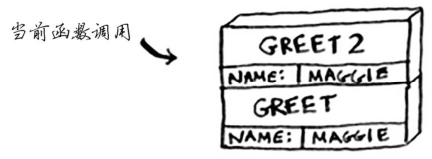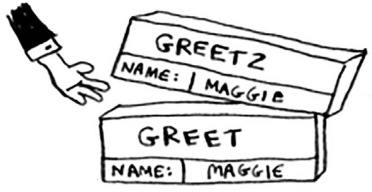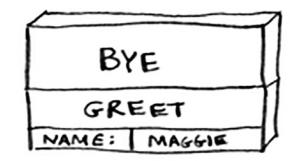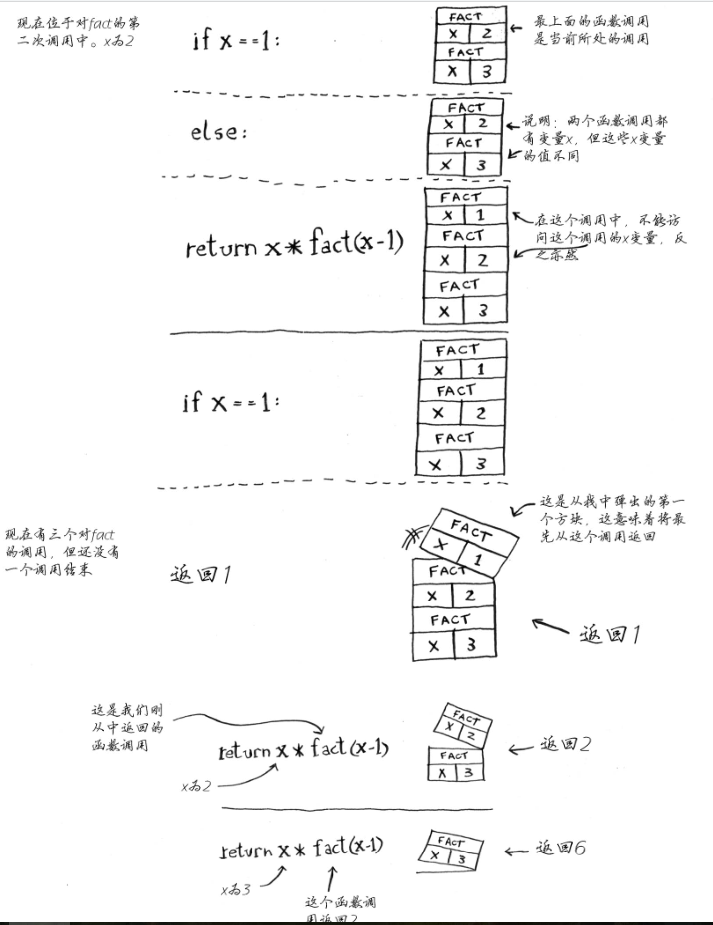# 递归-自上而下的思想### zhujingdi1998@gmail.com

```def greet2(name):
print("how are you, " + name + "?")
def bye():
print("ok bye!")

def greet(name):
print("hello, " + name + "!")
greet2(name)
print("getting ready to say bye...")
bye()
greet('maggie')
``````def fact(x):
if x == 1:
return 1
else:
return x * fact(x-1)
a = fact(3)
print(a)
```

`else:return x * fact(x-1)`是递归条件。617.合并二叉树 给定两个二叉树，想象当你将它们中的一个覆盖到另一个上时，两个二叉树的一些节点便会重叠。

```    Tree 1                     Tree 2
1                         2
/ \                       / \
3   2                     1   3
/                           \   \
5                             4   7
```

```         3
/ \
4   5
/ \   \
5   4   7
```

```class Solution {
public TreeNode mergeTrees(TreeNode t1, TreeNode t2) {

TreeNode res = mergeTree(t1,t2);
return res;
}

public TreeNode mergeTree(TreeNode t1,TreeNode t2){
if(t1==null)
return t2;
if(t2==null)
return t1;
t1.val +=t2.val;
t1.left= mergeTrees(t1.left,t2.left);
t1.right = mergeTrees(t1.right,t2.right);
return t1;

}
}
```